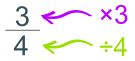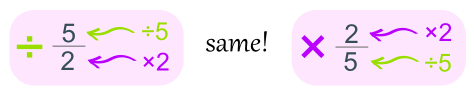# Dividing Fractions

Turn the second fraction upside down, then multiply.

## There are 3 Simple Steps to Divide Fractions:

 Step 1. Turn the second fraction (the one you want to divide by) upside down (this is now a reciprocal). Step 2. Multiply the first fraction by that reciprocal Step 3. Simplify the fraction (if needed)

### Example: 1 2   ÷   1 6

Step 1. Turn the second fraction upside down (it becomes a reciprocal):

1 6 becomes 6 1

Step 2. Multiply the first fraction by that reciprocal:

(multiply tops ...)

1 2  ×  6 1   =   1 × 6 2 × 1   =   6 2

(... multiply bottoms)

Step 3. Simplify the fraction:

6 2   =  3

### With Pen and Paper

And here is how to do it with a pen and paper (press the play button):

To help you remember:

"Dividing fractions, as easy as pie,
Flip the second fraction, then multiply.
And don't forget to simplify,
Before it's time to say goodbye"

 Another way to remember is: "leave me, change me, turn me over"### How Many?

20 divided by 5 is asking "how many 5s in 20?" (=4) and so:

1 2   ÷   1 6 is really asking:

how many 1 6 s in 1 2 ?

Now look at the pizzas below ... how many "1/6th slices" fit into a "1/2 slice"?

 How manyin? Answer: 3

So now you can see why 1 2   ÷   1 6 = 3

In other words "I have half a pizza, if I divide it into one-sixth slices, how many slices is that?"

### Another Example: 1 8   ÷   1 4

Step 1. Turn the second fraction upside down (the reciprocal):

1 4   becomes   4 1

Step 2. Multiply the first fraction by that reciprocal:

1 8   ×   4 1 = 1 × 4 8 × 1 = 4 8

Step 3. Simplify the fraction:

4 8   =   1 2

## Fractions and Whole Numbers

What about division with fractions and whole numbers?

Make the whole number a fraction, by putting it over 1.

Example: 5 is also 5 1

Then continue as before.

### Example: 2 3   ÷  5

Make 5 into 5 1 :

2 3   ÷   5 1

Then continue as before.

Step 1. Turn the second fraction upside down (the reciprocal):

5 1 becomes 1 5

Step 2. Multiply the first fraction by that reciprocal:

2 3 × 1 5 = 2 × 1 3 × 5 = 2 15

Step 3. Simplify the fraction:

The fraction is already as simple as it can be.

Answer = 2 15

### Example: 3  ÷   1 4

Make 3 into 3 1 :

3 1   ÷   1 4

Then continue as before.

Step 1. Turn the second fraction upside down (the reciprocal):

1 4 becomes 4 1

Step 2. Multiply the first fraction by that reciprocal:

3 1 × 4 1 = 3 × 4 1 × 1 = 12 1

Step 3. Simplify the fraction:

12 1 = 12

### And Remember ...

You can rewrite a question like "20 divided by 5" into "how many 5s in 20"

So you can also rewrite "3 divided by ¼" into "how many ¼s in 3" (=12)

### Why Turn the Fraction Upside Down?

Because dividing is the opposite of multiplying!

 A fraction says to: multiply by the top number divide by the bottom numberBut for DIVISION we:

• divide by the top number
• multiply by the bottom number

### Example: dividing by 5/2 is the same as multiplying by 2/5So instead of dividing by a fraction, it is easier to turn that fraction upside down, then do a multiply.